International
Tables for
Crystallography
Volume H
Powder diffraction
Edited by C. J. Gilmore, J. A. Kaduk and H. Schenk

International Tables for Crystallography (2018). Vol. H, ch. 3.9, pp. 350-353

## Section 3.9.4. Demonstration of methods

I. C. Madsen,a* N. V. Y. Scarlett,a R. Kleebergb and K. Knorrc

aCSIRO Mineral Resources, Private Bag 10, Clayton South 3169, Victoria, Australia,bTU Bergakademie Freiberg, Institut für Mineralogie, Brennhausgasse 14, Freiberg, D-09596, Germany, and cBruker AXS GmbH, Oestliche Rheinbrückenstr. 49, 76187 Karlsruhe, Germany

### 3.9.4. Demonstration of methods

| top | pdf |

The sample 1 suite from the IUCr Commission on Powder Diffraction (CPD) round robin on QPA (Madsen et al., 2001) provides a useful basis for demonstrating the applicability some of the methods described above. Sample 1 was designed to provide a relatively simple analytical system in order to determine the levels of accuracy and precision that could be expected under ideal conditions. The key design criteria required that the phases exhibit little peak overlap in the low-angle region of the diffraction pattern and the samples have at least one freestanding peak for each phase in the d-spacing range 3.7 to 1.9 Å.

The three components (corundum, α-Al2O3; fluorite, CaF2; and zincite, ZnO) were prepared in a ternary design to provide a total of eight different mixtures in order to cover as wide a range of composition as possible for each phase. The result is that each phase is present in the suite with concentrations of approximately 1, 4, 15, 33, 55 and 95 wt%. The exact compositions (Madsen et al., 2001) can be found in Table 3.9.1. The unique chemical composition of the component phases also allowed the weighed compositions to be confirmed by measurement of total elemental composition using X-ray fluorescence (XRF) methods.

 Table 3.9.1| top | pdf | Weighed composition (weight fraction) of the eight mixtures comprising sample 1 in the IUCr CPD round robin on QPA (Madsen et al., 2001)
SampleCorundumFluoriteZincite
1A 0.0115 0.9481 0.0404
1B 0.9431 0.0433 0.0136
1C 0.0504 0.0136 0.9359
1D 0.1353 0.5358 0.3289
1E 0.5512 0.2962 0.1525
1F 0.2706 0.1772 0.5522
1G 0.3137 0.3442 0.3421
1H 0.3512 0.3469 0.3019

Data sets were collected from three replicates of the eight mixtures using a Philips X'Pert diffractometer equipped with a Cu long fine focus tube operated at 40 kV and 40 mA. The beam path was defined with 1° divergence, 0.3 mm receiving and 1° scatter slits. A curved graphite post-diffraction monochromator was fitted to eliminate Kβ radiation. Data were collected from 15 to 145° 2θ in increments of 0.02° using a counting time of 1.5 s per step. These data sets are available as supporting information from http://it.iucr.org/ for any reader wishing to develop and test their skills in various methods.

For the single-peak methods, the net intensity for all peaks in the range 22 to 65° 2θ was extracted using a fundamental-parameters approach to peak fitting coded in the TOPAS software package (Bruker AXS, 2013). The choice of peak profile type is important, since any misfit will be reflected in the estimation of peak area and hence in the QPA. Unless otherwise stated, QPA was undertaken using the strongest peak in the pattern for each phase (corundum 113, d = 2.085 Å; fluorite 022, d = 1.932 Å; zincite 011, d = 2.476 Å). The average values for these peaks can be found in Table 3.9.2. For those methods requiring knowledge of the mass absorption coefficient,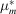for each sample was calculated from the XRF chemical analysis results.

 Table 3.9.2| top | pdf | Average values (n = 3) of net peak intensity derived using profile fitting for the strongest peaks of corundum (113), fluorite (022) and zincite (011)
 The figures in parentheses are the standard deviations of the means. The sample mass absorption coefficientwas calculated from the XRF-L determined composition.
SampleCorundumFluoriteZincite(cm2 g−1)
1A 34.8 (0.6) 8958.7 (33.0) 509.9 (6.0) 93.02
1B 6561.3 (28.6) 1095.5 (7.1) 474.3 (3.8) 34.45
1C 244.4 (0.9) 250.9 (10.1) 22898.0 (37.0) 49.03
1D 474.5 (3.5) 6559.6 (2.8) 5468.5 (9.5) 71.71
1E 2525.3 (27.9) 4835.5 (27.0) 3370.7 (16.3) 53.17
1F 1251.3 (7.8) 2935.8 (9.0) 12494.9 (22.4) 52.67
1G 1295.0 (8.7) 5041.7 (17.0) 6787.9 (26.6) 59.64
1H 1436.5 (7.3) 5132.0 (13.6) 5996.8 (59.5) 59.10

#### 3.9.4.1. Absorption–diffraction method

| top | pdf |

In this method, the QPA of each phase is conducted independently of the others. For each phase, the determination of a specific calibration constant, C, was achieved using a rearranged equation (3.9.3). The sample where the relevant phase was present at about 55 wt% (sample 1E for corundum, 1D for fluorite and 1F for zincite) was taken to be the calibration sample.

For fluorite the determination of C proceeded using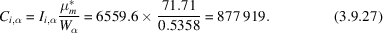All data sets were then analysed using equation (3.9.3), as demonstrated here using sample 1H.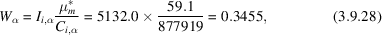compared with a value of 0.3469 added to the sample by weight. Fig. 3.9.1shows the analysed concentration for all 24 fluorite measurements along with the bias from the known values. The bias (analysed − known) all fall within the range −0.3 to 0.5 wt% with no systematic bias as a function of concentration. The similar results achieved for corundum and zincite demonstrate the validity of the approach where there is minimal peak overlap.Figure 3.9.1 | top | pdf |Plot of the analysed concentration (black diamonds – left axis) and the bias (open triangles – right axis) expressed as wt% for fluorite using the absorption–diffraction method. The analysis was calibrated using sample 1D, which has a fluorite concentration of 53.58 wt%.

#### 3.9.4.2. Internal standard method

| top | pdf |

Application of the internal standard method normally requires the addition of an appropriate phase in known amount to each sample to be analysed. In order to use this data for demonstration of the internal standard method, it is necessary to designate one of the existing phases as the internal standard. Sample 1H has been used to derive the calibration constant, with fluorite considered to be the phase of interest while zincite is designated as the internal standard. The intensities (Table 3.9.2) and known concentrations (Table 3.9.1) of these phases can then be used to derive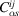from equation (3.9.8)to eliminate the need to know or measurefor the sample.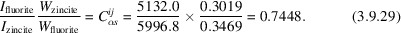Analysis of the unknowns (Fig. 3.9.2) then proceeds via equation (3.9.9)and is demonstrated here using sample 1D: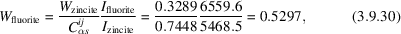compared with a value of 0.5358 added to the sample by weight.Figure 3.9.2 | top | pdf |Plot of the analysed concentration (black diamonds – left axis) and the bias (open triangles – right axis) expressed as wt% for fluorite using the internal standard method with zincite designated as the internal standard. The analysis was calibrated using sample 1H where the fluorite and zincite concentrations are 34.69 and 30.19 wt%, respectively.

#### 3.9.4.3. Reference intensity ratio

| top | pdf |

For this exercise, the peak intensities and phase concentrations in Tables 3.9.1and 3.9.2for sample 1H can be used to determine the RIRs for fluorite and zincite.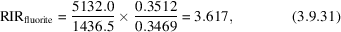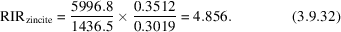These RIRs should be compared with reported values for fluorite in the ICDD database (ICDD, 2015) which have an average of 3.83 (n = 33) but range from 2.40 to 4.21. For zincite the reported RIR values have an average of 5.24 (n = 50) and range from 4.50 to 5.87. The discrepancies in the various reported values of the RIRs highlight the need to determine them under the same conditions as the samples being analysed if the highest accuracy is to be achieved.

Fig. 3.9.3shows the RIR values calculated from all 24 (eight samples, three replicates each) measurements for fluorite and zincite plotted as a function of corundum concentration. At intermediate concentrations there is quite good agreement between the determined values. However, there are significant deviations at low corundum concentration, resulting in insufficient measured intensity in the corundum peak to ensure sufficient accuracy in the RIR. Hence, care should be taken to ensure that there are sufficient counts in the peaks used to determine the RIR. In addition, a low concentration automatically means that there are fewer grains contributing to the diffraction process; hence particle statistics may also present a significant problem.Figure 3.9.3 | top | pdf |Plot of the 24 determined RIR values for fluorite (black diamonds) and zincite (open triangles) as a function of corundum concentration. The dashed lines represent the average RIR values for fluorite (lower) and zincite (upper) determined from the three replicates of sample 1H where all phases have approximately equal concentration.

The presence of other sample-related aberrations that affect the measured intensities also needs to be considered. For example, microabsorption may affect measured RIR values differently in different concentration ranges. The impact of such effects on the analysis is reduced by their inclusion in the measured RIR provided that variation induced by, for example, sample preparation can be kept to a minimum.

#### 3.9.4.4. Matrix flushing

| top | pdf |

Once the correct value of RIR is determined for each phase, the matrix-flushing method can be applied using equation (3.9.15). For fluorite in sample 1D, the calculation proceeds as follows: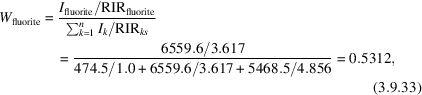compared with a value of 0.5358 added to the sample by weight. Fig. 3.9.4shows the bias for fluorite in all samples analysed by the matrix-flushing method. Once again, there is good agreement between the weighed and analysed amounts. However, it is worth reiterating that this method normalizes the sum of all analysed weight fractions to unity. If amorphous or non-analysed phases are present in the sample, then the weight fractions will be overestimated relative to their absolute abundances.Figure 3.9.4 | top | pdf |Plot of the analysed concentration (black diamonds – left axis) and the bias (open triangles – right axis) expressed as wt% for fluorite using the matrix-flushing method with RIRs of 1.0, 3.617 and 4.856 for corundum, fluorite and zincite, respectively. The RIRs were determined using sample 1H where the corundum, fluorite and zincite concentrations are 35.12, 34.69 and 30.19 wt%, respectively.

#### 3.9.4.5. Rietveld-based methods

| top | pdf |

The strengths and weaknesses of some of the methods described in Section 3.9.3are highlighted through a study of the mechanism and kinetics of nucleation and crystal growth in the context of the Bayer process for the extraction of aluminium from bauxite ores (Webster et al., 2010). Specifically, the experiments utilize synthetic Bayer liquors, consisting of Al-loaded caustic solutions to which a variety of seed material is added. Several polymorphs of Al(OH)3 (gibbsite, bayerite and nordstrandite) crystallize from solution onto the seed material. The rate of crystallization and the ratio of the phases formed depend on the sample conditions used, including the Al and caustic concentrations in solution, as well as sample temperature.

The mechanism and rate of crystallization were followed by collecting XRD data at the powder-diffraction beamline of the Australian Synchrotron4 over a period of about 3 h. The diffractometer incorporates a Mythen detector (Schmitt et al., 2003) which allows for the simultaneous collection of 80° 2θ of the diffraction pattern. A wavelength of 0.826 Å was used to ensure adequate penetration of the beam in the sample. The sample environment (Madsen et al., 2005; Norby et al., 1998) consisted of a 1-mm quartz glass capillary containing a slurry of the seed and Bayer liquor heated to temperatures between 333 and 348 K using a hot-air blower.

The data were analysed using TOPAS (Bruker AXS, 2013), where a learned-profile approach to peak modelling was used with an empirical instrument width and shape contribution determined using the NIST SRM660 LaB6 profile standard. For the samples in the study, refined parameters included 2θ zero offset, a Chebychev polynomial pattern background and, for each phase, the Rietveld scale factor, crystallite size and strain, and unit-cell dimensions.

A number of different approaches were used to extract the phase abundances at each stage of the reaction. Initially, QPA was derived using equation (3.9.26); the value that many Rietveld analysis programs output as their first estimate of phase abundance. Fig. 3.9.5shows the QPA output from an in situ experiment in which goethite (FeOOH) was added as the seed.Figure 3.9.5 | top | pdf |The results of QPA of the in situ XRD data collected during the seeding experiments of Webster et al. (2010). The values were derived using the Hill/Howard (Hill & Howard, 1987) relationship in equation (3.9.26). Note the decrease in apparent goethite concentration (left axis) as the polymorphs of Al(OH)3 (right axis) crystallize from solution.

At the start of the experiment, prior to the crystallization of any of the Al(OH)3 polymorphs, Fig. 3.9.5shows that the reported concentration of the goethite seed is 100 wt% since it is the only phase represented in the analysis at that time. On formation of gibbsite, bayerite and nordstrandite, the goethite concentration appears to decrease progressively to about 65 wt% while the total Al(OH)3 concentration reaches about 35 wt% at the end of the experiment. However, these figures are in disagreement with (i) the fact that goethite is unlikely to dissolve or otherwise be consumed in this system (Murray et al., 2009), (ii) the known addition of goethite to the sample (14.13 wt%) and (iii) the total amount of Al(OH)3 available from solution (15.92 wt%). The problem with the QPA in this case arises from the fact that only the crystalline components are considered in the analysis and that equation (3.9.26)normalizes the sum of their analysed weight fractions to unity. However, aluminium, which is in solution at the start of the run, forms crystalline phases continuously throughout the reaction after an initial induction period. In order to overcome the anomalies in the QPA results, it is necessary to consider the sample as a whole; that is, the concentration of both the solid and liquid components in the X-ray beam for the duration of the experiment.

In this sample, the concentration of the goethite seed was 14.13 wt% in the slurry injected into the sample capillary. If the assumption is made that, in this environment, goethite is unreactive and its concentration will not change during the reaction, it can be used as an internal standard to put the Al(OH)3 concentrations on an absolute basis. The QPA results derived using the internal standard or spiked' approach in equation (3.9.25)are shown in Fig. 3.9.6.Figure 3.9.6 | top | pdf |The results of QPA of the in situ XRD data collected during the seeding experiments of Webster et al. (2010).The values are absolute phase abundances derived using the internal standard relationship in equation (3.9.25).

The goethite concentration is fixed at the known addition (14.13 wt%) at the start of the experiment. However, the concentrations of the Al(OH)3 polymorphs are now put on an absolute scale, thus allowing derivation of more meaningful reaction mechanisms.

If, however, there is residual doubt about the reactivity of the goethite, it may be necessary to use the external standard approach embodied in equation (3.9.21). In this case, the value for the instrument constant, K, can be derived using the Rietveld scale factor, ZMV and the known addition of goethite in a rearranged equation (3.9.21). For this determination, the goethite scale factor from the first few data sets, prior to the start of the reaction, was averaged to minimize any errors that may be introduced by counting statistics. The value of the sample mass absorption coefficientwas set to an arbitrary value of unity for both the determination of K and all subsequent analyses, since the overall chemical content of the capillary, and hence the attenuation of the X-ray beam, does not change during the reaction.

This experimental work was conducted at the Australian Synchrotron where the storage-ring current was boosted every 12 h. Between these times the current, and hence the incident-beam intensity, decays, resulting in what amounts to a change in the instrument configuration'. This requires a modification of the K value and subsequent calculation of concentration to compensate for the changing incident intensity using equation (3.9.22).

Fig. 3.9.7now shows the results of QPA derived from equation (3.9.22). In this case the concentrations of the Al(OH)3 polymorphs are similar to those in Fig. 3.9.6. However, since the phase abundances are derived using an external standard approach, any changes in the apparent goethite concentration can now be monitored. Fig. 3.9.7shows that the goethite concentration did not change significantly in the early stages of the experiment (t < 10 min) before Al(OH)3 crystallization was observed but there is a small, systematic decrease in the apparent goethite concentration as the experiment progresses. At the end of the experiment, the goethite concentration appears to be lower by about 1% relative to the concentration at the start.Figure 3.9.7 | top | pdf |The results of QPA of the in situ XRD data collected during the seeding experiments of Webster et al. (2010). The values are absolute phase abundances derived using the external standard relationship in equation (3.9.22). Note the slight decrease in the goethite concentration (left axis) during the run.

This apparent decrease could be due to a number of causes including (i) poor correction for beam-intensity changes or (ii) solid material moving about in the capillary with some movement out of the X-ray beam. Alternatively, the decrease could be attributed to the `shielding' of the goethite from the X-ray beam by the Al(OH)3 phases as they form and coat the goethite particles. This decrease could then be used to obtain an average thickness of the Al(OH)3 phases on the seed particles. This layer was calculated to be about 5.5 µm (assuming a linear absorption coefficient of 9.5 cm−1 for gibbsite at 0.826 Å) resulting in an overall particle size of about 11 µm at the end of the run (the goethite particles are about 0.2 × 2 µm and hence do not contribute significantly to the overall particle size). These values are in good agreement with independent studies (Webster et al., 2010) where the gibbsite was examined using scanning electron microscopy (SEM) techniques (Fig. 3.9.8) following crystallization under similar conditions to those used here.Figure 3.9.8 | top | pdf |SEM image of Al(OH)3 (grey hexagon) which has crystallized on goethite seed (light grey needles) (Webster et al., 2010).

### References

Bruker AXS (2013). Topas v5: General profile and structure analysis software for powder diffraction data. Version 5. https://www.bruker.com/topas.Google Scholar
ICDD (2015). The Powder Diffraction File, database of the International Centre for Diffraction Data, release PDF4+, 2015. ICDD, 12 Campus Boulevard, Newton Square, Pennsylvania 19073–3273, USA.Google Scholar
Madsen, I. C., Scarlett, N. V. Y., Cranswick, L. M. D. & Lwin, T. (2001). Outcomes of the International Union of Crystallography Commission on Powder Diffraction Round Robin on Quantitative Phase Analysis: samples 1a to 1h. J. Appl. Cryst. 34, 409–426.Google Scholar
Madsen, I. C., Scarlett, N. V. Y. & Whittington, B. I. (2005). Pressure acid leaching of nickel laterite ores: an in situ diffraction study of the mechanism and rate of reaction. J. Appl. Cryst. 38, 927–933.Google Scholar
Murray, J., Kirwan, L., Loan, M. & Hodnett, B. K. (2009). In-situ synchrotron diffraction study of the hydrothermal transformation of goethite to hematite in sodium aluminate solutions. Hydrometallurgy, 95, 239–246.Google Scholar
Norby, P., Cahill, C., Koleda, C. & Parise, J. B. (1998). A reaction cell for in situ studies of hydrothermal titration. J. Appl. Cryst. 31, 481–483.Google Scholar
Schmitt, B., Brönnimann, C., Eikenberry, E. F., Gozzo, F., Hörmann, C., Horisberger, R. & Patterson, B. (2003). Mythen detector system. Nucl. Instrum. Methods Phys. Res. A, 501, 267–272.Google Scholar
Webster, N. A. S., Madsen, I. C., Loan, M. J., Knott, R. B., Naim, F., Wallwork, K. S. & Kimpton, J. A. (2010). An investigation of goethite-seeded Al(OH)3 precipitation using in situ X-ray diffraction and Rietveld-based quantitative phase analysis. J. Appl. Cryst. 43, 466–472.Google Scholar